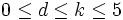# Congruence condition on number of abelian subgroups of small prime power order and bounded exponent for odd prime

Jump to: navigation, search
This article is about a congruence condition.
View other congruence conditions
This article defines a replacement theorem
View a complete list of replacement theorems| View a complete list of failures of replacement

## Statement

### In terms of universal congruence condition

Suppose$p$ is an odd prime, and$0 \le d \le k \le 5$. Then, the collection of abelian groups of order$p^k$ and exponent dividing$p^d$ is a Collection of groups satisfying a universal congruence condition (?).

### Hands-on statement

Suppose$p$ is an odd prime, and$P$ is a finite$p$-group. Suppose$0 \le d \le k \le 5$. Suppose$P$ has an abelian subgroup of order$p^k$ and exponent dividing$p^d$. Then, the following equivalent statements hold:

1. The number of abelian subgroups of$P$ of order$p^k$ and exponent dividing$p^d$ is either equal to zero or congruent to$1$ modulo$p$.
2. The number of abelian normal subgroups of$P$ of order$p^k$ and exponent dividing$p^d$ is congruent to$1$ modulo$p$.
3. For any finite$p$-group$L$ containing$P$, the number of abelian subgroups of$P$ of order$p^k$ and exponent dividing$p^d$ that are normal in$L$ is congruent to$1$ modulo$p$.